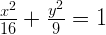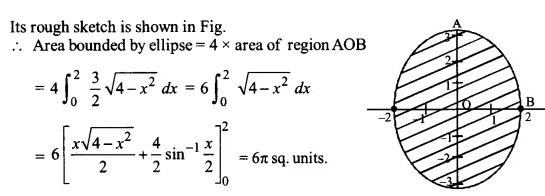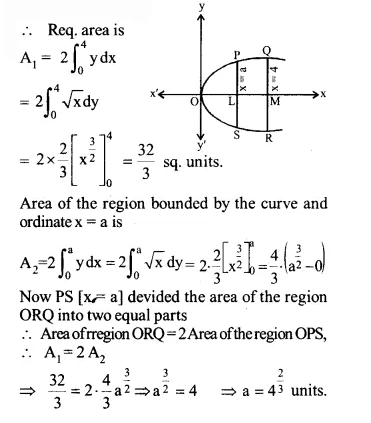NCERT Solutions for Maths Class 12 Exercise 8.1

# NCERT Solutions for Maths Class 12 Exercise 8.1

## NCERT Solutions for Maths Class 12 Exercise 8.1

Hello Students. Welcome to maths-formula.com. In this post, you will find the complete NCERT Solutions for Maths Class 12 Exercise 8.1.

You can download the PDF of NCERT Books Maths Chapter 9 for your easy reference while studying NCERT Solutions for Maths Class 12 Exercise 8.1.

Class 12th is a very crucial stage of your student’s life, since you take all important decisions about your career on this stage. Mathematics plays a vital role to take decision for your career because if you are good in mathematics, you can choose engineering and technology field as your career.

NCERT Solutions for Maths Class 12 Exercise 8.1 helps you to solve each and every problem with step by step explanation which makes you strong in mathematics.

All the schools affiliated with CBSE, follow the NCERT books for all subjects. You can check your syllabus from NCERT Syllabus for Mathematics Class 12.

NCERT Solutions for Maths Class 12 Exercise 8.1 are prepared by the experienced teachers of CBSE board. If you are preparing for JEE Mains and NEET level exams, then it will definitely make your foundation strong.

If you want to recall All Maths Formulas for Class 12, you can find it by clicking this link.

If you want to recall All Maths Formulas for Class 11, you can find it by clicking this link.

NCERT Solutions for Maths Class 12 Exercise 8.2

## NCERT Solutions for Maths Class 12 Exercise 8.1

Maths Class 12 Ex 8.1 Question 1.

Find the area of the region bounded by the curve y² = x and the lines x = 1, x = 4 and the x-axis.

Solution:

The curve y² = x is a parabola with vertex at the origin. The x-axis is the line of symmetry, which is the axis of parabola. The area of the region bounded by the curve, x = 1, x = 4 and the x-axis.

Maths Class 12 Ex 8.1 Question 2.

Find the area of the region bounded by y² = 9x, x = 2, x = 4 and the x-axis in the first quadrant.

Solution:

The given curve is y² = 9x, which is a parabola with vertex at the origin and the axis along x-axis. It is symmetrical about x-axis, as it contains only even powers of y. x = 2 and x = 4 are straight lines parallel to y-axis at a positive distance of 2 and 4 units from it, respectively.

Maths Class 12 Ex 8.1 Question 3.

Find the area of the region bounded by x² = 4y, y = 2, y = 4 and the y-axis in the first quadrant.

Solution:

The given curve x² = 4y is a parabola with vertex at the origin. Also, since it contains only even powers of x, it is symmetrical about y-axis. y = 2 and y = 4 are straight lines parallel to x-axis at a positive distance of 2 and 4 from it, respectively.

Maths Class 12 Ex 8.1 Question 4.

Solution:

The equation of the ellipse isThe given ellipse is symmetrical about both the axes as it contains even powers of x and y both.

Maths Class 12 Ex 8.1 Question 5.

Solution:

It is an ellipse with centre (0, 0) and the length of major axis = 2a = 2 × 3 = 6 and the length of minor axis = 2b = 2 × 2 = 4.Maths Class 12 Ex 8.1 Question 6.

Find the area of the region in the first quadrant enclosed by x-axis, line x = √3y and the circle x² + y² = 4.

Solution:

Consider the two equations x² + y² = 4           … (i)
and x = √3y, i.e. y = x/√3           … (ii)
(1) x² + y² = 4 is a circle with centre O(0, 0) and radius = 2.

Maths Class 12 Ex 8.1 Question 7.

Find the area of the smaller part of the circle x² + y² = a² cut off by the line x = a/√2.

Solution:

The equation of the given curves are x² + y² = a²      … (i)

and x = a/√2   … (ii)

Clearly, (i) represents a circle and (ii) is the equation of a straight line parallel to y-axis at a distance of a/√2 units to the right of y-axis.

Maths Class 12 Ex 8.1 Question 8.

The area between x = y² and x = 4 is divided into two equal parts by the line x = a, find the value of a.

Solution:

The graph of the curve x = y² is a parabola as given in the following figure. Its vertex is O and the axis is x-axis. QR is the ordinate along x = 4.Maths Class 12 Ex 8.1 Question 9.

Find the area of the region bounded by the parabola y = x² and y = |x|.

Solution:

Clearly, x² = y represents a parabola with vertex at (0, 0) in the positive direction of y-axis as its axis opens upwards.
y = |x|, i.e., y = x and y = –x represent two lines passing through the origin and making an angle of 45° and 135° with the positive direction of the x-axis.
The required region is the shaded region as shown in the following figure. Since both the curve are symmetrical about y-axis.

So, the required area = 2 (shaded area in the first quadrant)

Maths Class 12 Ex 8.1 Question 10.

Find the area bounded by the curve x² = 4y and the line x = 4y – 2

Solution:

The given curve is a x² = 4y             … (i)
which is an upward parabola with vertex at (0, 0) and it is symmetrical about y-axis
Equation of the line is x = 4y – 2     … (ii)
Solving (i) and (ii) simultaneously, we get (4y – 2)² = 4y
⇒ 16y² – 16y + 4 = 4y
⇒ 4y² – 5y + 1 = 0

Maths Class 12 Ex 8.1 Question 11.

Find the area of the region bounded by the curve y² = 4x and the line x = 3.

Solution:

The curve y² = 4x is a parabola as shown in the following figure. The axis of the parabola is x-axis. The area of the region bounded by the curve y² = 4x and the line x = 3 is
A = Area of region OPQ = 2 (Area of the region OLQ)

Choose the correct answer in the following Exercises 12 and 13.

Maths Class 12 Ex 8.1 Question 12.

Area lying in the first quadrant and bounded by the circle x² + y² = 4 and the lines x = 0 and x = 2 is
(A) π
(B) π/2
(C) π/3
(D) π/4

Solution:

(A) The given curve is x² + y² = 4. It is a circle with the centre at (0, 0) and r = 2.

Maths Class 12 Ex 8.1 Question 13.

Area of the region bounded by the curve y² = 4x, y-axis and the line y = 3 is
(A) 2
(B) 9/4
(C) 9/3
(D) 9/2

Solution:

(B) The given curve is y² = 4x, which is a parabola.